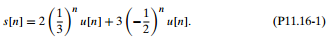### Write the normal equations for this case

Assignment Help Chemical Engineering
##### Reference no: EM131140142

Consider the signalWe wish to model this signal using a 2nd-order (p = 2) all-pole model or, equivalently, using 2nd-order linear prediction. For this problem, since we are given an analytical expression fors[n] and s[n] is the impulse response of an all-pole filter, we can obtain the linear prediction coefficients directly from the z-transform of s[n]. (You are asked to do this in part (a).) In practical situations, we are typically given data, i.e., a set of signal values, and not an analytical expression. In this case, even when the signal to be modeled is the impulse response of an all-pole filter, we need to perform some computation on the data, using methods such as those discussed in Section 11.3, to determine the linear prediction coefficients. There are also situations in which an analytical expression is available for the signal, but the signal is not the impulse response of an all-pole filter, and we would like to model it as such. In this case, we again need to carry out computations such as those discussed in Section 11.3.

(a) For s[n] as given in Eq. (P11.16-1), determine the linear prediction coefficients a1, a2 directly from the z-transform of s[n].

(b) Write the normal equations for p = 2 to obtain equations for a1, a2 in terms ofrss[m].

(c) Determine the values of rss, rss, and rss for the signal s[n] given in Eq. (P11.16-1).

(d) Solve the system of equations from part (a) using the values you found in part (b) to obtain values for the aks

(e) Are the values of ak from part (c) what you would expect for this signal? Justify your answer clearly. (f) Suppose you wish to model the signal now with p = 3. Write the normal equations for this case.

(g) Find the value of rss. (h) Solve for the values of ak when p = 3. (i) Are the values of ak found in part (h) what you would expect given s[n]? Justify your answer clearly. (j) Would the values of a1, a2 you found in (h) change if we model the signal with p = 4?

#### Calculate the chemical potential of methanol in solution

Calculate the chemical potential of methanol in solution with acetic acid at xMeOH = 0.8, 80 °C, 0.4 bar. The reference state is the pure liquid at 80 °C, 0.4 bar.

#### What are the major provisions of the epcrtka

For what reasons did congress enact the Emergency Planning Community Right to Know Act? How is this Act related to OSHA, RCRA, SARA, and CERCLA? What are the major provision

#### Aqueous sulfuric solutions containing

Aqueous sulfuric solutions containing 15.0 wt% H2SO4 and 80.0 wt% H2SO4 are mixed to form a 30.0 wt% product solution. The 15 % solution was in a lab in which the temperature

#### Find the volume of the plasma compartmen

Suppose 500 mg of dye was introduced into the plasma compartment. After reaching steady state, the concentration in the blood is 0:0893 mg cm3. Find the volume of the plasma

#### Find the line current delivered by the source

Balanced wye-connected loads drawing 10 kW at 0.8 power factor lagging and 15 kW at 0.9 power factor leading are connected in parallel and supplied by a 60-Hz, 300-V, three-

#### Determine the values of vmax

Determine the values of Vmax, KM, and KI. v, M/min x 106 S, M No inhibitor 10-5 M inhibitor 3.3 x 10-4 56 37 5.0 x 10-4 71 47 6.7 x 10-4 88 61 1.65 x 10-3 129 103 2.21 x 10-

A 10-hp, 250-V shunt motor has an armature circuit resistance of 0.5 Ω and a field resistance of 200 Ω. At no load, rated voltage, and 1200 r/min, the armature current is 3

#### Calculate the concentration of all ions

Calculate the concentration of all ions present in each of the following solutions of strong electrolytes. a. 0.0200 mol of sodium phosphate in 10.0 mL of solution b. 0.300

### Write a Review